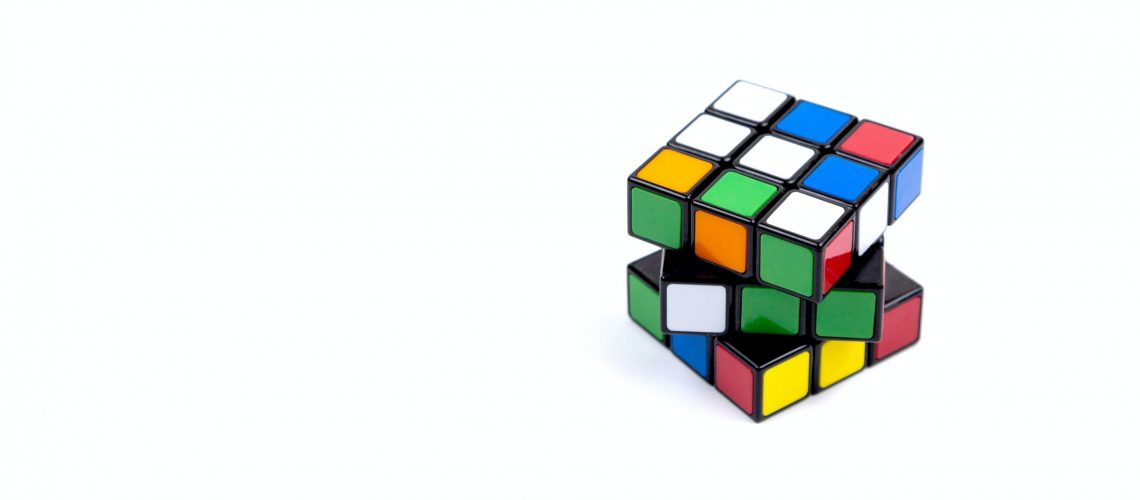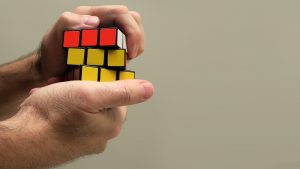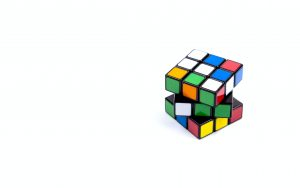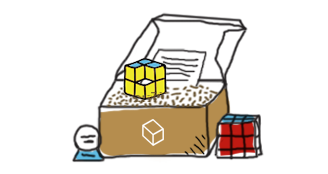# Rubik’s Cube Solving in 20 Moves: God’s Number

Many puzzle-loving people have been stumped by the Rubik’s cube and its frustrating ability to remain unsolved. Fellow puzzlers will agree that after hours of turning the scrambled cube in every direction, it may seem like only God can solve it!

In fact, this is closer to the truth than puzzlers realize. God’s number refers to the number 20, the maximum number of moves it takes to solve the quintillions of combinations(permutations) of the cube.

Of course, actually solving the cube in 20 moves would involve a god-like brain capable of sifting through millions of combinations in seconds – hence the term God’s number. The number 20 has also been linked to research done by top academics and mathematicians who believe that it is the key to explaining life as we know it and the entire universe.

Solving the Rubik’s cube can be done by following a precise sequence of moves to get the colored cubes into the correct position. This seems relatively simple, but with more than 490 million possible combinations, it may seem best left to the professional speedcubers.

However, many cubers start with the beginner’s method, a series of moves and algorithms that unscrambles the cube until a white cross is formed on the cube face. This is the starting point from which each corner piece and edge piece is matched to the centerpieces of each cube face.

Solving the Rubik’s cube is a highly competitive puzzle sport. With many competing to hold the world record for the fastest cube solution. This title is currently held by Yusheng Du, who can solve the cube in a mere 3.47 seconds.

## History of God’s Number

God’s number was researched for the first time in 1981 by Morwen Thistlewaite. Using a complex algorithm, he proved that the 43 quintillion possible cube positions could be solved in 52 moves.

The maximum number of moves required to achieve a Rubik’s cube solution gradually reduced over the years to 29 in the 1990s and then went down to 20 after googling supercomputers were used to assist with the research in the early 2000s.

The research was conducted by a team of mathematicians and Tomas Rokicki, who working with Google, developed an algorithm that could be used to test millions of cube positions.

In July 2010, their research proved God’s number to be 20. Google provided the processing power needed to run the algorithm, which took a few weeks. If the average home PC had been used for this process, it would have taken approximately 35 years!

However, the actual invention of the Rubik’s cube goes back to 1974. The cubes founding father, Erno Rubik, a professor of architecture, developed it as a type of 3D geometry model.

Solving the Rubik’s cube involves a series of moves that solvers must follow step by step to solve the cube. These are also known as move count metrics or turn metrics, which count the number of moves it takes for solvers to find the cube solution.

The half-turn metric is also called the face turn metric, where turning any one of the cubes’ faces counts as one move. While the quarter-turn metric is when any cube face is moved by 90 degrees either clockwise or anti-clockwise, this counts as one move.## The Super-Flip

The super-flip has its roots entrenched in the work of programmer and mathematician Herbert Kociemba author of Cube Explorer. He invented the Kociemba Algorithm in 1992, improving on the Thistlethwaite Algorithm. But it wasn’t until 2010 that he and Tomas Rokicki finally set the upper bounds of the cube at 20 – Gods number.

The super-flip itself was originally discovered by Michale Reid in 1995. This configuration is also known as the 3x3x3 position. This is when the cubes’ corners, edges, and center cubies are in the correct solved position but are flipped. To achieve this pattern, solvers need to complete 20 moves or half turn metrics (HTM). The super flip was the first position discovered that could not be solved using less than 20 moves.

Cubers can use the below sequence of clockwise and counterclockwise moves to achieve the super-flip position. However, before we dive into how to do this, beginner cubers need to get to grips with some basic concepts.

We all know that cube algorithms (patterns) use a series of letters to understand which cube face requires moving. However, knowing which one to turn to can be tricky. When cubing the cube movements or face rotations are described by letters.

For example:

F (front), B (back), R (right), L (left), D (down), U (up)

Single uppercase letters mean the cube face should be turned clockwise by 90- degrees. While letters followed by an apostrophe indicate a counterclockwise turn of 90 degrees is required. This is also known as an inverted turn. Finally, letters with the number 2 after them mean the cuber should turn the face twice by 180-degrees.

Now that we have the basics out the way, the below sequence of moves can be used to solve the superflip position.

R L U2 F U’ D F2 R2 B2 L U2 F’ B’ U R2 D F2 U R2## God’s Number for 2x2x2

The 2x2x2 is a simpler version of the Rubik’s cube and is often called the Pocket Cube or Mini Cube. Eight smaller cubes make up the 2x2x2 puzzle, and each face can rotate, rearranging the four smaller cubes at that face. The puzzle is equivalent to only the corners of a regular Rubik’s cube, and there are no face centers.

The 2x2x2 has eight pieces, and each has three orientations, giving a maximum of 8!.3^8(eight factorial multiplied by three to the power of eight) positions. However, you can’t reach the limit because the cube has a total fixed twist or rotations of three, and the puzzle’s orientation doesn’t matter. Therefore, only 7!·3^6 (seven factorial multiplied by three to the power of 6) = 3,674,160 positions or permutations remain.

Many cube solvers have used computer searches to find God’s algorithm for the 2x2x2 from as far back as the 1980s. The results have proved God’s Number as 11 moves using the half-turn metric or 14 movements using the quarter-turn metric if you consider half a turn to be two moves.

You can solve the puzzle in three phases. The first phase involves solving the top layer in the following steps:

•  Decide the color you want on the top face and hold the cube so that at least one piece shows that color on the top face.
• Find a piece in the D layer that belongs in the U layer. Rotate D by displacing any wrong piece in the U layer.
• Depending on the orientation, do one of the following:
• To move FRD ->URF, do FDF’
• To move RDF -> URF, do R’D’R
•  To move DFR -> URF, do FD’F’R’D2R

Repeat this sequence until you complete the top layer.

For phase two, rotate the bottom layer D to get at least two pieces in the correct position, even if they’re twisted.

You can swap two pieces if necessary using the following algorithm:

Do F’R’D’RDFD’ to swap DFR, DBL
Do FDF’D’R’D’R swap DFR, DFL

The third phase involves correctly twisting the bottom layer pieces to complete and solve the puzzle. The following sequences can help solve the remaining portions.

Note that a + denotes clockwise twists while a – indicates anti-clockwise twists:

• To twist DFL-, DBL+  do R’D’R F’DR’DRD2F2
• To twist DFL+, DBL-  do F2D2 R’D’RD’ R’DR
• To twist DFL-, DBR+  do R2D’R D2R’D2R D’R2D
• To twist DFR-, DRB-, DBL-  do R’D’RD’ R’D2RD2
• To twist DFR+, DRB+, DBL+  do D2R’D2R DR’DR
• To twist DFR-, DRB+, DBL-, DLF+  do R2D2 R D2R2 D
• To twist DFR+, DRB-, DBL-, DLF+  do RDF R2D2F2 DF’DR2

## God’s Number for 4x4x4

There hasn’t been any confirmed God’s number for the 4x4x4 cube or higher. However, there are various inconsistencies since the centerpiece isn’t fixed. God’s number for the 4x4x4 is estimated to be between 30 and 33. Through the outer block turn metric (OBTM), God’s number for 4x4x4 is known to be between 35 and 55 moves.

In the single slice turn metric (SSTM), God’s number is between 32 and 53 moves, while in the block turn metric (BTM), it’s known to be between 29 and 53 movements. Since no definitive confirmation is available, you should expect more discoveries in the future!